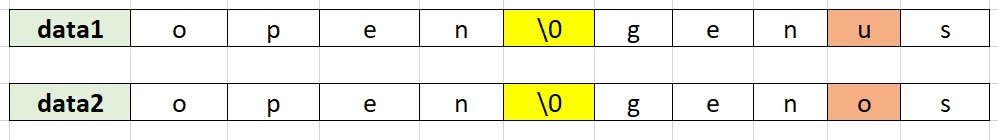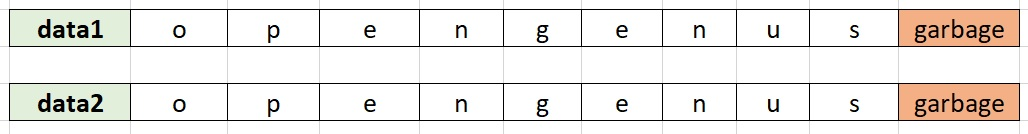×

Search anything:

# strcmp in C

#### Software Engineering C ProgrammingIndian Technical Authorship Contest starts on 1st July 2023. Stay tuned.

Reading time: 30 minutes | Coding time: 10 minutes

strcmp is a function in C which is used to compared two strings that is array of characters and returns if the first array is greater, smaller or equal to the second array in form of integer. It is a part of string.h header file. Hence, to use this function, include the header file as:

``````#include <string.h>
``````

Usage:

``````strcmp(array1, array2);
``````

Note:

• It will return an integer (0 for equal, negative for array1 < array2, positive for array1 > array2)
• array1 and array2 are pointers to same or different character array

Major points/ limitations:

• Comparison continues until a different character is found or null character (\0) is found in any of the strings
• the major issue is buffer overflow due to the above point
• It does not compare null characters or beyond it.

We have explained all points in detail using examples.

In our first example, we will create two strings or character pointers having the same data and use strcmp function on it. Complete example:

``````#include <stdio.h>
#include <string.h>

int main()
{
char* data1 = "this is data1";
char* data2 = "this is data1";
printf("%d", strcmp(data1, data2));
return 0;
}
``````

Output:

``````0
``````

Note that in the above example, C automatically adds a null character \0 to the end of both strings.

We will look at another example where the first data is greater than the second data. In this example, we will set data1 to the string "this is data2" and see what will be the output.

``````#include <stdio.h>
#include <string.h>

int main()
{
char* data1 = "this is data2";
char* data2 = "this is data1";
printf("%d", strcmp(data1, data2));
return 0;
}
``````

Output:

``````1
``````

Note that even though data1 is greater than data2, the output can be any number greater than 0 (not necessary 1). This is because of the way strcmp is implemented internally. It simply subtracts the ASCII value of characters to perform the comparison.

Consider this example where we have set data1 to the string "this is data5" and the answer should be 4 as 5 - 1 is equal to 4 even in ASCII.

``````#include <stdio.h>
#include <string.h>

int main()
{
char* data1 = "this is data5";
char* data2 = "this is data1";
printf("%d", strcmp(data1, data2));
return 0;
}
``````

Output:

``````4
``````

Now, we will consider an example in which data2 is greater. For this, we will set data2 to the string "this is data99" and data1 stays as "this is data1". The expected output should be -8 as 1-9 is -8.

Consider this C code:

``````#include <stdio.h>
#include <string.h>

int main()
{
char* data1 = "this is data1";
char* data2 = "this is data95";
printf("%d", strcmp(data1, data2));
return 0;
}
``````

Output:

``````-8
``````

Note that in the above example, the last character of data2 that is 5 is never checked as the previous character is establishes the difference.

Hence, the point is:

Once a character is found to be different, further characters are not checked.

## Limitation of strcmp

Another point is that comparison is also stopped when '\0' character is encountered in any of the strings. This character denotes the end of the string. This is a limitation.

One trick is that even is the two strings are different, strcmp can return that both are same if the null character is encountered for both strings at the same location before the different characters are encountered.

Consider this data:Note that the strings are different (note u and o) but there is a null character in between and before that all characters are same.

Consider this example:

``````#include <stdio.h>
#include <string.h>

int main()
{
char data1[] = {'o', 'p', 'e', 'n', '\0', 'g', 'e', 'n', 'u', 's'};
char data2[] = {'o', 'p', 'e', 'n', '\0', 'g', 'e', 'n', 'o', 's'};
printf("%d", strcmp(data1, data2));
return 0;
}
``````

Output:

``````0
``````

Even though both array are different, it is returning that both are same. The only way to overcome this limitation is to use your own implementation for comparison.

## Issue of strcmp

Building on the previous idea, another problem is that is the null character is not present. In this case, the comparison will continue indefinitely and will access memory locations outside the array and hence, it will read garbage value due to which output will be different everytime.

Similarly, consider this data:Note that in this case, both data are same (opengenus) but we have not added the null character so strcmp does not know when to stop comparing. Due to this, it goes beyond the array values and compare garbage values which gives wrong results.

Consider this code where the strings are same but we have not given the null character:

``````#include <stdio.h>
#include <string.h>

int main()
{
char data1[] = {'o', 'p', 'e', 'n', 'g', 'e', 'n', 'u', 's'};
char data2[] = {'o', 'p', 'e', 'n', 'g', 'e', 'n', 'u', 's'};
printf("%d", strcmp(data1, data2));
return 0;
}
``````

Output:

``````-4
``````

Output changes everytime.

Hence, the leasons learnt about using strcmp in C are:

• Do not use strcmp if you want to compare beyond null characters
• Do not use strcmp if your data does not have null characters

With this, you have the complete knowledge to use strcmp as a master C programmer. Enjoy.#### OpenGenus Tech Review Team

The official account of OpenGenus's Technical Review Team. This team review all technical articles and incorporates peer feedback. The team consist of experts in the leading domains of Computing.